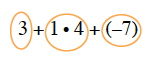### Home > CC2 > Chapter 4 > Lesson 4.2.4 > Problem4-63

4-63.

Circle terms and simplify each of the following expressions. Homework Help ✎

1. $3+1·4+(−7)$

Begin by circling each term.Simplify the expression.

$3+4+(−7)$
$7+(−7)=0$

$0$

2. $50+3(−10)+2(−20)$

Following the same steps as in part (a), try solving this problem before checking the answer.

$−20$

3. $4+(−50)·2$

Follow the same steps as in part (a). Make sure you are circling the terms, so that simplifying is easier.

4. $(4+(−170))(5+(−4))$

In this problem, make sure to add within the parentheses before multiplying for the final product.